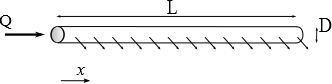# Distributor pipe

Analytical relationship for the direct calculation of pressure drops in pipes distributing a flow rate in a homogeneous manner based on the Blasius formula.

## AssumptionsWe assume a pipe length $$L$$, inner diameter $$D$$, with a flow rate at the top $$Q$$. We calculate the pressure drop between the two ends of the pipe. In a constant flow section $$q$$, the friction coefficient is evaluated with the Blasius formula, valid for moderate Reynolds numbers for smooth walls:

$\lambda \simeq a Re^{-0.25}$

## Analytical development

We're recording the position from the downstream end of the pipe. The flow rate is supposed to vary linearly with $$x$$, and is then written:

$q(x)=Q x/L$

Let's note $$S=\pi D^2/4$$ the inner surface of the pipe. The pressure drop is obtained by integrating the Darcy-Weisbach relationship:

$\Delta H=\int_{x=0}^{L} a Re^{-0.25} \frac{u^2(x)}{2gD}dx$

Note the kinematic viscosity. We then replace $$Re$$ with $$u D/\nu$$, which gives

$\Delta H=\int_{x=0}^{L} a u(x)^{-0.25}D^{-0.25}\nu ^{0.25} \frac{u^2(x)}{2gD}dx$

By rearranging, we get:

$\Delta H=\int_{x=0}^{L} a \nu ^{0.25} \frac{u^{1.75}(x)}{2gD^{1.25}}dx$

Let's use the flow equation to show the flow ($$u(x)=q(x)/S$$):

$\Delta H=\int_{x=0}^{L} a \nu ^{0.25} \frac{(Qx/(LS))^{1.75}}{2gD^{1.25}}dx$

then the diameter:

$$\(\Delta H=\int_{x=0}^{L} a \nu ^{0.25} \frac{(4Qx/(L\pi D^2))^{1.75}}{2gD^{1.25}}dx$$\) We rearrange to get

$\Delta H=a \nu ^{0.25} \frac{(4/\pi)^{1.75}Q^{1.75}}{2g D^{4.75}} \int_{x=0}^{L} (x/L)^{1.75}dx$

By integrating, we obtain

$\Delta H=a \nu ^{0.25} \frac{(4/\pi)^{1.75}Q^{1.75}}{2g D^{4.75}}\frac{L}{2.75}$
$\Delta H=a \nu ^{0.25} \frac{4^{1.75}}{5.5g \pi^{1.75}}{Q^{1.75}L}{D^{4.75}}$

## Digital application

For water at 20°C: $$\nu\simeq 10^{-6}$$ m2/s, which gives

$Delta H=0.323\ 10^{-3}\frac{Q^{1.75}}{D^{4.75}}L$

with $$\Delta H$$ in meters.

For water at 50°C, $$\nu\simeq 0.556 10^{-6}$$ m2/s, which means that the pressure drop is reduced by about 14%, or

$Delta H= 0.28\ 10^{-3}\frac{Q^{1.75}}{D^{4.75}}L$